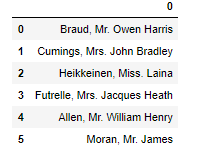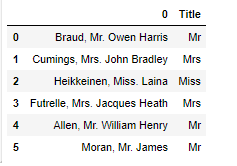# 3 Advanced Python Features You Should Know

As a Data Scientist, you are already spending most of your time getting your data ready for prime time. Follow these real-world scenarios to learn how to leverage the advanced techniques in Python of list comprehension, Lambda expressions, and the Map function to get the job done faster.Photo by David Clode on Unsplash.

In this article, I will discuss 3 important features of Python that comes in handy for a data scientist and saves a lot of time. Let’s begin without wasting any time.

### List & Dict Comprehensions

List & Dict Comprehensions are a very powerful tool in Python that comes in handy to make lists. It saves time, easy in syntax, and makes the logic easier as compared to making a list using normal Python for-loop.

Basically, these are used instead of an explicit for-loop with simple logic inside it that appends or does something with a list or dictionary. These are single line and makes the code more readable.

List Comprehensions

Will will see a normal python example first and then will see it’s equivalent using list comprehensions.

Scenario

Let’s say we have a simple dataframe in Pandas with students results in 3 subjects.

```In : results = [[10,8,7],[5,4,3],[8,6,9]]

In : results = pd.DataFrame(results, columns=['Maths','English','Science'])

```

And if we print it,

```In : results

Out:
Maths  English  Science
0     10        8        7
1      5        4        3
2      8        6        9

```

Now let’s say we want to make a list with maximum marks of each subject, i.e., that list should be equal to [10,8,9] because these are maximum marks of each subject.

Python Code

```In : maxlist = []

In : for i in results:
...:     maxlist.append(max(results[i]))

```

And, if we print it,

```In : maxlist

Out: [10, 8, 9]

```

Using List Comprehensions

The basic syntax for a list comprehension is

```[varname for varname in iterableName]

```

Here we can do anything with varname to modify it.

In our case, our list comprehension would be

```In :maxlist = [max(results[i]) for i in results]

```

And our output would be

```Out: [10, 8, 9]

```

Do you see how it resembles our Python code? We were appending max(results[i]) in maxlist inside the loop in normal Python code, here we are not appending it, but anything you want to append should the first argument in the list comprehension. So now I can rewrite the basic syntax as

```newlist = [whatYouwantToAppend for anything in anyIterable]

```

Hence, in our examples, we wanted to append the maximum of each row in a dataframe, i.e., max(results[i]) so we used

```[max(results[i]) for i in results]

```

Dictionary Comprehension

Similarly, we use the same idea for dictionary comprehension. Basic syntax normally used is

```{key, value for key, value in anyIterable}

```

For example, let’s say that I have a dummy table for the English alphabet,

```df =
{0: 'A',
1: 'B',
2: 'C',
3: 'D',
4: 'E',
5: 'F',
6: 'G',
7: 'H',
8: 'I',
9: 'J',
10: 'K',
11: 'L',
12: 'M',
13: 'N',
14: 'O',
15: 'P',
16: 'Q',
17: 'R',
18: 'S',
19: 'T',
20: 'U',
21: 'V',
22: 'W',
23: 'X',
24: 'Y',
25: 'Z'}

```

Now, if we want to use numbers (0–25) as our values of the dictionary because a machine learning model can only process numbers, and we want to feed the dictionary values, w need to swap the keys and values of a dictionary. The easiest way to swap the keys and values in the dictionary is using dict comprehension.

```{number: alphabet for alphabet , number in df.items()}

```

If you focus on it for a second, it will start making sense that how it is reversing a dictionary, that we are looping alphabet and number which is the normal order of dictionary but what we want to insert is in reverse order so we are using number:alphabet instead of alphaber:number.

### Lambda Expressions

Lambda Expressions are a very powerful tool for data scientists. They come in very handy especially when used with DataFrame.apply(), map(), filter() or reduce(). They provide an easy way to avoid manually defined functions and to write them in a single line making code more clearer.

Let’s see the basic syntax of Lambda Expression, where we return the next number of any number passed in any function.

```z = lambda functionArgument1 : functionArgument1 + 1

```

Here, our function argument is functionArgument1, and we are returning functionArgument1 +1 which is the next number.

So to use it,

```z(5)
>>>6

```

See, it is that simple. Let’s see a real-world example where we have a dataframe of names (taken from the titanic dataset), and we want to make a feature where we want to extract the title (Mr, Miss, Sir etc.) from the name.

So, the code for our data frame is

```df = pd.DataFrame([
'Braud, Mr. Owen Harris',
'Heikkeinen, Miss. Laina',
'Futrelle, Mrs. Jacques Heath',
'Allen, Mr. William Henry',
'Moran, Mr. James'
])

```

and the dataframe isNow, if we notice that we find a specific pattern here, which is that we have a comma after the first name and ‘full stop’ after the title that we want to extract. So we can use list slicing to extract from the comma until the full stop.

We want to slice from 2 indexes next to a comma because we don't want to include a comma and space after comma so our starting index will be str.find(',')+2, and we want to slice to the full stop (not included), so we will slice until str.find('.'). Luckily, the last index is not included, so we do not need to do -1 explicitly from the index. Let’s use a lambda expression to solve this problem.

```df['Title'] = df.apply(lambda a: a[a.find(',')+2: a.find('.')])

```

Here we are using the .apply function from pandas on df, which is the column having all the names. Inside it, we are passing a lambda expression that takes in each name and returns the sliced version of that name. This sliced version is the title of that name. The output of our dataframe now isAnd here, we can see that how we extracted the titles from the name using this pretty clear trick.

Lambda expressions are very useful when applied with mapreduce or filter in Python.

### Map Function

In Python, Map functions are very commonly used functions, which makes our work very easier. The idea of map is that when passed a function and an iterable in map, it performs that function on every single entity of that iterable.

What does it mean?

Let’s say that I have an iterable that is a list with the following data [0, 5, 10, 15, 20, 25, 30], and a custom function isEven(anyInteger) that tells if anyInteger passed into it is even or not. If we pass this function and the iterable into map, then it will automatically apply this function to all the entities in the list and return the map object, which we can convert into a list or tuple or dictionary.

Example

Let’s say our function is

```def isEven(anyInteger):
return anyInteger % 2 == 0

```

and our iterable is

```myList = [0, 5, 10, 15, 20, 25, 30]

```

If we want to use the traditional approach, then we would have to loop our list and apply the function to individual items.

```# Traditional approach
isEvenList = []
for i in myList:
isEvenList.append(isEven(i))

```

This will return us a new list isEvenList with having True for Even and False for odd numbers.

To use it with map it will make our task much easier. Our code would be

```isEvenList = list(map(isEven, myList))

```

and our code is done!

Here, what we are doing is that inside the map function, we are passing in our function isEven and our iterable myList, which is returning a map object which we are casting into list.

So basic syntax for the map function is

```list/dict/tuple(map(myFunction, myIterable))

```

Luckily, Pandas offer mapapply, and applymap as built-in functions. They are very commonly used and are very helpful. The basic idea behind all these 3 functions is the same as Python’s built-in map function. You can learn more about these functions in the official documentation of Pandas here.

Related: